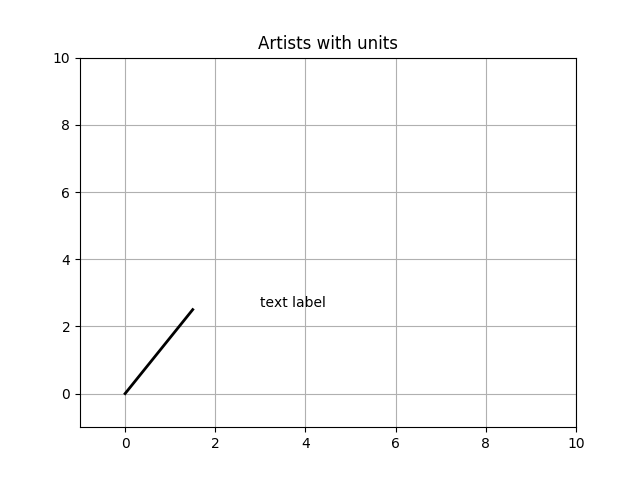Artist tests¶

Test unit support with each of the Matplotlib primitive artist types.

The axis handles unit conversions and the artists keep a pointer to their axis parent. You must initialize the artists with the axis instance if you want to use them with unit data, or else they will not know how to convert the units to scalars.

This example requires basic_units.pyimport random
import matplotlib.lines as lines
import matplotlib.patches as patches
import matplotlib.text as text
import matplotlib.collections as collections

from basic_units import cm, inch
import numpy as np
import matplotlib.pyplot as plt

fig, ax = plt.subplots()
ax.xaxis.set_units(cm)
ax.yaxis.set_units(cm)

# Fixing random state for reproducibility
np.random.seed(19680801)

if 0:
# test a line collection
# Not supported at present.
verts = []
for i in range(10):
# a random line segment in inches
verts.append(zip(*inch*10*np.random.rand(2, random.randint(2, 15))))
lc = collections.LineCollection(verts, axes=ax)

# test a plain-ol-line
line = lines.Line2D([0*cm, 1.5*cm], [0*cm, 2.5*cm],
lw=2, color='black', axes=ax)

if 0:
# test a patch
# Not supported at present.
rect = patches.Rectangle((1*cm, 1*cm), width=5*cm, height=2*cm,
alpha=0.2, axes=ax)